## Green's Function--Helmholtz Differential Equation

The inhomogeneous Helmholtz Differential Equation is(1)

where the Helmholtz operator is defined as. The Green's function is then defined by(2)

Define the basis functions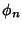as the solutions to the homogeneous Helmholtz Differential Equation(3)

The Green's function can then be expanded in terms of thes,(4)

and the Delta Function as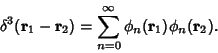(5)

Plugging (4) and (5) into (2) gives(6)

Using (3) gives(7)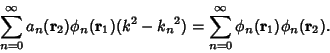(8)

This equation must hold true for each, so(9)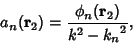(10)

and (4) can be written(11)

The general solution to (1) is therefore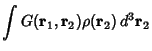(12)

References

Arfken, G. Mathematical Methods for Physicists, 3rd ed. Orlando, FL: Academic Press, pp. 529-530, 1985.